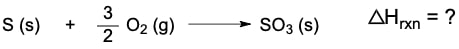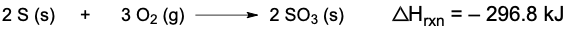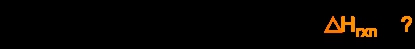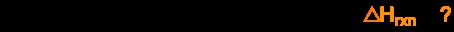Start typing, then use the up and down aroows to select an option from the list.

# GOB Chemistry

Learn the toughest concepts covered in your GOB - General, Organic, and Biological Chemistry class with step-by-step video tutorials and practice problems.

Energy, Rate and Equilibrium

Hess's Law involves the use of partial reactions in the determination of the overall enthalpy of reaction.

## Hess's Law

1

### concept

Hess's Law3m
Play a video:
0
2

### example

Hess's Law Example 11m
Play a video:
0
3
Problem

Calculate the ∆Hrxn for the following thermochemical equation:When given the following:4

### concept

Hess's Law2m
Play a video:
0
5

### example

Hess's Law Example 25m
Play a video:
0
6
Problem

Calculate the ∆Hrxn forGiven the following set of reactions:7
Problem

Calculate the ∆Hrxn forGiven the following reactions: